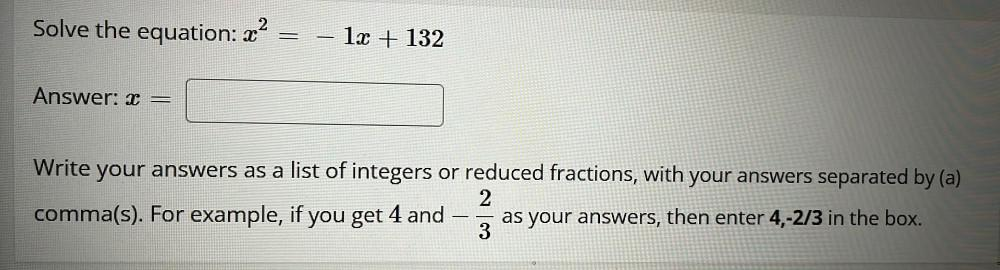Question:

# x^2=-1x +132 x = Write your answers as a list of integers or reduced fractions, with your answers separated by (a) comma(s). For example, if you get 4 and -2/3 as your answers, then enter 4,-2/3 inx^2=-1x +132 x = Write your answers as a list of integers or reduced fractions, with your answers separated by (a) comma(s). For example, if you get 4 and -2/3 as your answers, then enter 4,-2/3 in the box.